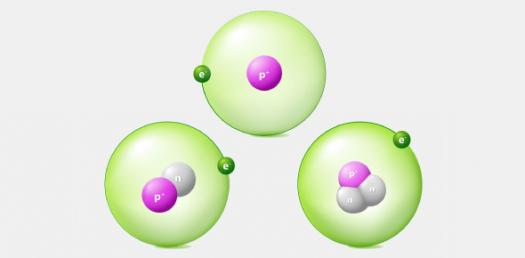15 Questions | Attempts: 241SettingsThis trivia quiz is designed to Test Your Knowledge about Atoms, Ions and Isotopes! As a science student how much do you know about the interrelation between these three and how they are formed. The quiz below is specifically designed to help you refresh your memory, do give it a shot and see if it actually works. All the best!

• 1.
Protons...
• A.

Are negatively charged

• B.

Orbit the nucleus

• C.

Are positively charged

• D.

1/2000 amu

• 2.
Electrons...
• A.

Orbit the nucleus and negatively charged

• B.

Are positively charged and make up the nucleus

• C.

Take up most of the mass in the atom

• D.

Are the same as the atomic number

• 3.
The nucleus
• A.

Is made up of protons and neutrons

• B.

Orbits the center proton

• C.

Is the coldest point in the atom

• D.

Surrounds the atom

• 4.
The atomic number
• A.

Tells what can and what cannot move in an atom

• B.

Orbits the center proton

• C.

States how many spoolas are in the nucleus

• D.

Is the same as the amount of protons

• 5.
AMU stands for
• A.

Absolute Mass Unit

• B.

Atomic Making Unit

• C.

Atomic Mass Unit

• D.

Abraham's Mass Unit

• 6.
If the atomic number is 9, the number of protons is
• A.

8

• B.

9

• C.

1

• D.

12

• 7.
If Uranium's atomic number is is 92 and it has 10 neutrons, how many protons does it have?
• A.

92

• B.

86

• C.

82

• D.

102

• 8.
If Aluminum has an atomic number of 13 and has 14 neutrons, what is its mass?
• A.

25

• B.

1

• C.

32

• D.

27

• 9.
An ion forms when an atom gains or losses.
• A.

Protons.

• B.

Neutrons.

• C.

Electrons.

• D.

Quarks.

• 10.
Two atoms that have different numbers of protons are
• A.

Different ions.

• B.

Different isotopes.

• C.

Different elements.

• 11.
Area around an atomic nucleus where an electron is most likely to be found
• A.

Electron cloud

• B.

Nucleus

• C.

Proton

• 12.
1. Which of the following elements in the chart is a cation (positive charge)? [Blank]
2. Which of the following elements in the chart is anion (negative charge)? pick one [Blank]
3. Which of the following elements in the chart is a stable atom? [Blank]
Calcium (Ca) Beryllium (Be) Fluorine (F) Neon (Ne) Protons 20 4 9 10 Neutrons 21 5 9 10 Electrons 20 5 10 9
• 13.
1. Here are three isotopes of an element:            C-12   C-13   C-14
The atomic number is 6.
1. The element is: [Blank]
1. The numbers 12, 13, and 14 refer to the[Blank]
2. How many protons [Blank]and neutrons[Blank] are in the first isotope?
3. How many protons[Blank] and neutrons[Blank] are in the second isotope?
4. How many protons[Blank] and neutrons[Blank] are in the third isotope?

## Related TopicsBack to top
×

Wait!
Here's an interesting quiz for you.